## Jordan Curve Theorem, Proof# JCT - Simple Cases

Scott E. Brodie, MD, PhD
Icahn School of Medicine at Mount Sinai
New York

• JCT holds for circles; for ellipses; for triangles

• Indeed, JCT holds for any "star-shaped" region whose boundary is a Jordan curve.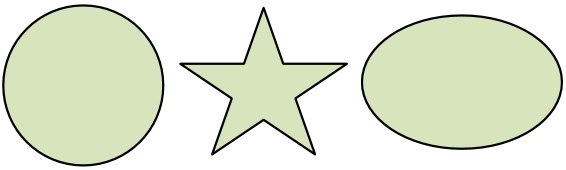In particular, JCT holds for every convex region whose boundary is a Jordan curve.

### Jordan separation for polygons: any polygon separates the plane

• Proof:

• For each point in the complement of the polygon, define a parity function by drawing a ray to a point outside a circle which contains the entire polygon in its interior, and counting the number of intersections between the ray and the polygon: the parity is odd or even as the number of intersections is odd or even.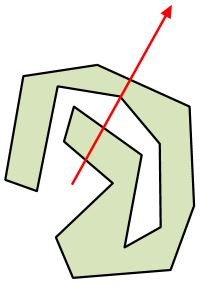• Fine print: ignore rays which intersect a vertex of the polygon (including rays with segments of the polygon as subsets).

• Consistency

One must show consistency: rays extending from the same point in different directions yield the same parity: rotate one such ray towards another – the intersection count can change only as the ray crosses a vertex.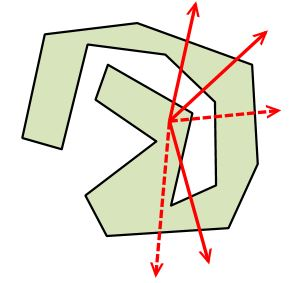If the two segments which meet at the vertex lie on the same side of the ray, the intersection count changes by two; if the two segments lie on opposite sides of the ray, the intersection count does not change – in either case, the parity remains constant.

• Points connected by a polygonal arc which does not meet the polygon have the same parity: slide a ray along a segment of the polygonal arc, preserving its orientation. As above, the intersection count can change only as the ray crosses a vertex, but at each vertex, parity is conserved.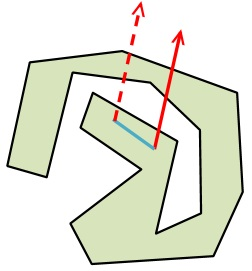• Conversely, points of the same parity may be connected by a polygonal arc which does not intersect the original polygon:

• draw a straight segment from one such point to another. If this segment does not intersect the polygon, we are done. If it intersects the polygon only at one or more vertices, one or more small polygonal detours will make it disjoint from the polygon, and we are again done.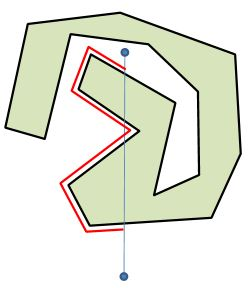• Otherwise, this transversal must intersect the polygon an even number of times, and can intersect each segment of the polygon at most once. Starting from a point of the transversal just before the first intersection with the polygon, trace a new polygonal arc along the polygon (never crossing it) until a point just beyond the last intersection point is reached. [This is possible because one can specify a minimal distance between the portion of the polygon intercepted by the connecting line, and remaining segments of the polygon, and keep even closer to the polygon while tracing the new connecting polygonal arc, and because the transversal separates the each segment of the polygon which it intersects.]

• From this last point, the last portion of the connecting segment completes the polygonal arc between the points. As nowhere does the new polygonal arc intersect the original polygon, parity is preserved.

• Separation: draw a small circle centered on an interior point of any segment of the polygon, which does not contain any vertices of the polygon. The segment divides the circle into two regions, which must have opposite parity. Thus there exist points of both odd and even parity.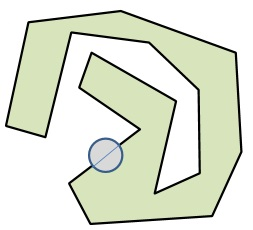• The sets of odd and even parity are thus nonempty, disjoint, open, and connected. That is, the polygon separates the plane.

• Note that each segment of a Jordan polygon forms part of the boundary of exactly two components.

### The unbounded component of the complement is unique.

• The complement of a compact set (for example, a Jordan curve, a Jordan arc, a Jordan polygon, or polygonal arc) contains exactly one unbounded component (as any two such components must both intersect the exterior of a suitably large circle).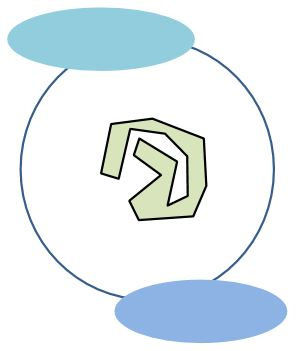• The unbounded component is the exterior of the set. The union of the bounded components, if any, constitutes the interior of the set.## Jordan Curve Theorem, Proof